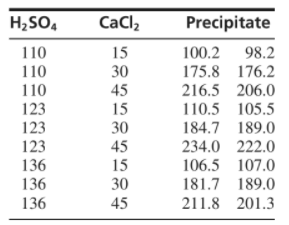×
Get Full Access to Statistics For Engineers And Scientists - 4 Edition - Chapter 9 - Problem 21se
Get Full Access to Statistics For Engineers And Scientists - 4 Edition - Chapter 9 - Problem 21se

×

# Solved: The article “Factorial Experiments in theISBN: 9780073401331 38

## Solution for problem 21SE Chapter 9

Statistics for Engineers and Scientists | 4th Edition

• Textbook Solutions
• 2901 Step-by-step solutions solved by professors and subject experts
• Get 24/7 help from StudySoup virtual teaching assistantsStatistics for Engineers and Scientists | 4th Edition

4 5 1 340 Reviews
27
0
Problem 21SE

The article “Factorial Experiments in the Optimization of Alkaline Wastewater Pretreatment” (M. Prisciandaro, A. Del Borghi, and F. Veglio, Industrial Engineering and Chemistry Research, 2002:5034–5041) presents the results of an experiment to investigate the effects of the concentrations of sulfuric acid (H2SO4) and calcium chloride (CaCl2) on the amount of black mud precipitate in the treatment of alkaline wastewater. There were three levels of each concentration, and two replicates of the experiment were made at each combination of levels. The results are presented in the following table (all measurements are in units of kg/m3).a. Construct an ANOVA table. You may give ranges for the P-values.

b. Is the additive model plausible? Explain.

c. Can you conclude that H2SO4 concentration affects the amount of precipitate? Explain.

d. Can you conclude that CaCl2 concentration affects the amount of precipitate? Explain.

Step-by-Step Solution:
Step 1 of 3

Chemistry of Solutions Lecture no. 7 Thursday, September 15, 2016 Topics Covered: Le Châtelier’s principle, the nature of acids and bases, acid strength, the pH scale, and calculating the pH of strong acid solutions. Le Châtelier's Principle If a change is imposed on a system at equilibrium, the position of the equilibrium will shift in a direction that tends to reduce that change. A chemical reaction is not a sentient being, if a substance is added the rates of the forward and reverse reactions are disturbed. The net reaction occurs in one direction, until the system reaches equilibrium again, At the new equilibrium position, the reactants and products have different concentrations (compared to their initial concentrations). 1. Changing co

Step 2 of 3

Step 3 of 3

##### ISBN: 9780073401331

Statistics for Engineers and Scientists was written by and is associated to the ISBN: 9780073401331. This full solution covers the following key subjects: concentration, explain, amount, precipitate, alkaline. This expansive textbook survival guide covers 153 chapters, and 2440 solutions. This textbook survival guide was created for the textbook: Statistics for Engineers and Scientists , edition: 4. The full step-by-step solution to problem: 21SE from chapter: 9 was answered by , our top Statistics solution expert on 06/28/17, 11:15AM. The answer to “?The article “Factorial Experiments in the Optimization of Alkaline Wastewater Pretreatment” (M. Prisciandaro, A. Del Borghi, and F. Veglio, Industrial Engineering and Chemistry Research, 2002:5034–5041) presents the results of an experiment to investigate the effects of the concentrations of sulfuric acid (H2SO4) and calcium chloride (CaCl2) on the amount of black mud precipitate in the treatment of alkaline wastewater. There were three levels of each concentration, and two replicates of the experiment were made at each combination of levels. The results are presented in the following table (all measurements are in units of kg/m3). a. Construct an ANOVA table. You may give ranges for the P-values.b. Is the additive model plausible? Explain.c. Can you conclude that H2SO4 concentration affects the amount of precipitate? Explain.d. Can you conclude that CaCl2 concentration affects the amount of precipitate? Explain.” is broken down into a number of easy to follow steps, and 136 words. Since the solution to 21SE from 9 chapter was answered, more than 339 students have viewed the full step-by-step answer.

Unlock Textbook Solution